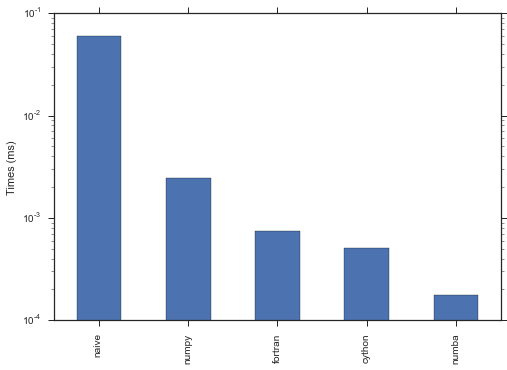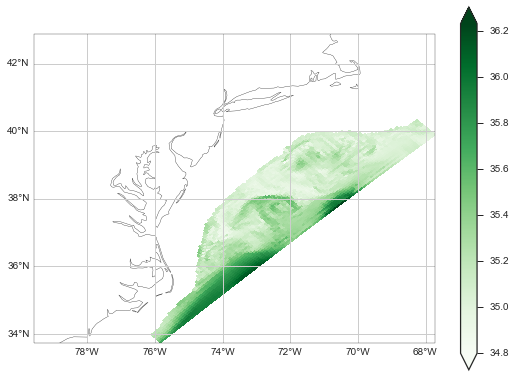In :
name = '2015-10-05-isosurfaces'
title = "Optimizing code for iso-surfaces using Fortran, Cython, and Numba"

In :
%matplotlib inline

import os
from datetime import datetime
from IPython.core.display import HTML

with open('creative_commons.txt', 'r') as f:

hour = datetime.utcnow().strftime('%H:%M')

date = '-'.join(name.split('-')[:3])
slug = '-'.join(name.split('-')[3:])

date=date,
hour=hour,
slug=slug,
name=name)

markdown = """Title: {title}
date:  {date} {hour}
slug: {slug}

{{% notebook {name}.ipynb cells[2:] %}}

content = os.path.abspath(os.path.join(os.getcwd(),
os.pardir,
os.pardir,
'{}.md'.format(name)))
with open('{}'.format(content), 'w') as f:
f.writelines(markdown)

html = '''
<small>
<p> This post was written as an IPython notebook.
<p></p>
%s''' % (name, name, html)


A very common operation for ocean modelers is to extract an iso-surface from 3D the model results. The application ranges from fancy isopycnals 3D views to simply getting any variable sliced at some arbitrary depth.

In this post I will explore a few different ways compute iso-surfaces. First we have to create some fake data for the experiment.

In :
import numpy as np

p = np.linspace(-100, 0, 30)[:, None, None] * np.ones((50, 70))
x, y = np.mgrid[0:20:50j, 0:20:70j]

q = np.sin(x) + p
p0 = -50.


In :
def naive_zslice(q, p, p0, mask_val=np.NaN):
N, M, L = q.shape, q.shape, q.shape

q_iso = np.empty((M, L))
for i in range(L):
for j in range(M):
for k in range(N-1):
if (((p[k, j, i] < p0) and (p[k+1, j, i] > p0)) or
((p[k, j, i] > p0) and (p[k+1, j, i] < p0))):
dp = p[k+1, j, i] - p[k, j, i]
dp0 = p0 - p[k, j, i]
dq = q[k+1, j, i] - q[k, j, i]
q_iso[j, i] = q[k, j, i] + dq*dp0/dp
return q_iso

In :
naive = %timeit -n1000 -o naive_zslice(q, p, p0)

1000 loops, best of 3: 59.9 ms per loop


It works, but it is way too slow. Imagine a slice of a high resolution global ocean model! The "state-of-art" for iso-surface is the original version, written in Fortran, that I based my naive_zslice() above.

Since can easily wrap Fortran in Python let's try that out.

In :
%load_ext fortranmagic

In :
%%fortran

subroutine fortran_zslice(q, p, p0, q_iso, L, M, N)
implicit none
integer L, M, N
real*8 q(N,M,L)
real*8 p(N,M,L)
real*8 q_iso(M,L)
cf2py intent(out) q_iso
integer i, j, k
real*8 dq, dp, dp0, p0

do i=1,L
do j=1,M
q_iso(j,i)=1.0d20 ! default value - isoline not in profile
do k=1,N-1
if ( (p(k,j,i).lt.p0.and.p(k+1,j,i).gt.p0).or.
&           (p(k,j,i).gt.p0.and.p(k+1,j,i).lt.p0) ) then
dp = p(k+1,j,i) - p(k,j,i)
dp0 = p0 - p(k,j,i)
dq = q(k+1,j,i) - q(k,j,i)
q_iso(j,i) = q(k,j,i) + dq*dp0/dp
endif
enddo
enddo
enddo

return
end subroutine fortran_zslice

In :
fotran = %timeit -n1000 -o fortran_zslice(q, p, p0)

1000 loops, best of 3: 746 µs per loop


An impressive speedup! Way to go good old Fortran. However, it is not easy to ship Python wrapped Fortran code on Windows :-(

We can be more Windows friendly and try Cython. Note that cython also needs a compilation step, but compiling cython code on Windows is way easier than Fortran.

In :
%load_ext Cython

In :
%%cython

cimport cython
import numpy as np
cimport numpy as np

@cython.boundscheck(False)
@cython.wraparound(False)
def cython_zslice(double[:, :, ::1] q,
double[:, :, ::1] p,
double p0,
cdef int L = q.shape
cdef int M = q.shape
cdef int N = q.shape
cdef double dp, dq, dq0
cdef int i, j, k

cdef double[:, ::1] q_iso = np.empty((M, L), dtype=np.float64)

for i in range(L):
for j in range(M):
for k in range(N-1):
if (((p[k, j, i] < p0) and (p[k+1, j, i] > p0)) or
((p[k, j, i] > p0) and (p[k+1, j, i] < p0))):
dp = p[k+1, j, i] - p[k, j, i]
dp0 = p0 - p[k, j, i]
dq = q[k+1, j, i] - q[k, j, i]
q_iso[j, i] = q[k, j, i] + dq*dp0/dp
return np.array(q_iso)

In :
cython = %timeit -n1000 -o cython_zslice(q, p, p0)

1000 loops, best of 3: 505 µs per loop


Slightly better than Fortran ;-) I am not a cython expert. All I did was follow to follow a few blog posts (see 1 and 2). I am pretty sure someone might come up with a way to make this faster.

Another possibility I wanted to try is the new kid on the block, numba.

I remember meeting Travis Oliphant during the AMS Meeting in 2012. At that time all he could talk about was llvm and some crazy ideas on how to use it.

A few months later those ideas became numba and, since then, numba has evolved a lot. One one side numba is still hard to install by yourself, but conda takes that pain away. (Available on Windows too BTW.)

The easiest way to use numba is to import the just-in-time compiler decorator and decorate your function. Note that numba is "kind-of" like the Julia language and pypy: it can optimize dumb loops, but if your code use some smart vectorization you won't get much out of it.

Here is a copy the naive_zslice from above decorated with numba.

In :
from numba.decorators import jit

@jit
N, M, L = q.shape, q.shape, q.shape

q_iso = np.empty((M, L))
for i in range(L):
for j in range(M):
for k in range(N-1):
if (((p[k, j, i] < p0) and (p[k+1, j, i] > p0)) or
((p[k, j, i] > p0) and (p[k+1, j, i] < p0))):
dp = p[k+1, j, i] - p[k, j, i]
dp0 = p0 - p[k, j, i]
dq = q[k+1, j, i] - q[k, j, i]
q_iso[j, i] = q[k, j, i] + dq*dp0/dp
return q_iso

In :
numba = %timeit -n1000 -o numba_zslice(q, p, p0)

1000 loops, best of 3: 175 µs per loop


A fantastic speedup with literally zero effort. I spent a few hours reading about cython and spoke with some people with more experience to get the results above. In numba all I did was to add the @jit decorator!

Just for the sake of completeness let's try using a few numpy tricks and make a non-naive Python version.

Note that this function was in fact one of my very first Python functions! It was based on an old Matlab script to do zslices.

In :
def numpy_zslice(q, p, p0):
N, L, M = q.shape

p0 = -abs(p0)

data = q.reshape(N, -1, order='F')
z = p.reshape(N, -1, order='F')

bottom = np.zeros((1, L*M))
top = np.empty_like(bottom)
top.fill(-np.inf)
z = np.r_[top, z, bottom]

top.fill(np.NaN)
data = np.r_[top, data, data[-1, ...][None, :]]

z, data = map(np.flipud, (z, data))

zg_ind = np.diff(z < p0, axis=0).ravel('F').nonzero()
zg_ind += np.arange(0, len(zg_ind), 1)
depth_greater_z = z.ravel('F')[zg_ind]
data_greater_z = data.ravel('F')[zg_ind]

zl_ind = np.diff(z > p0, axis=0).ravel('F').nonzero()
zl_ind += np.arange(1, len(zg_ind)+1, 1)
depth_lesser_z = z.ravel('F')[zl_ind]
data_lesser_z = data.ravel('F')[zl_ind]

alpha = (p0-depth_greater_z) / (depth_lesser_z-depth_greater_z)
data_at_depth = (data_lesser_z*alpha) + (data_greater_z*(1-alpha))
return data_at_depth.reshape(L, M, order='F')

In :
numpy = %timeit -n1000 -o numpy_zslice(q, p, p0)

1000 loops, best of 3: 2.46 ms per loop


Sure it is not as good as the compiled trio (Numba, Fortran, and Cython), but not bad either. Also, unlike the other options, numpy is easy to install and ship any where. (Including my old n900.)

Summarize the result in one graph:

In :
from pandas import Series

benchmarkings = dict(naive=naive.best,
numpy=numpy.best,
fortran=fotran.best,
cython=cython.best,
numba=numba.best)

benchmarkings = Series(benchmarkings)
benchmarkings.sort(ascending=False)

ax = benchmarkings.plot(kind='bar', logy=True)
yt = ax.set_ylabel('Times (ms)')And to close this post up let's try iso-slices with some real data.

In :
import warnings

import iris

url = ('http://tds.marine.rutgers.edu/thredds/dodsC/roms/espresso/2013_da/avg/'
'ESPRESSO_Real-Time_v2_Averages_Best')

with warnings.catch_warnings():
warnings.simplefilter("ignore")

In :
salt = cubes.extract_strict('sea_water_salinity')[-1, ...]  # Last time step.

lon = salt.coord(axis='X').points
lat = salt.coord(axis='Y').points

In :
p = salt.coord('sea_surface_height_above_reference_ellipsoid').points
q = salt.data

In :
p0 = -500.0

naive_500 = naive_zslice(q, p, p0)
numba_500 = numba_zslice(q, p, p0)
numpy_500 = numpy_zslice(q, p, p0)
fortran_500 = fortran_zslice(q, p, p0)
# I could not figure out how to use mixed float32/float64 as input for cython!
cython_500 = cython_zslice(q.astype(np.float64), p, p0)


And we have to to be sure to check if all these functions return the same result.

In :
import numpy.ma as ma

slices = naive_500, cython_500, numba_500, numpy_500
naive_500, cython_500, numba_500, numpy_500 = map(ma.masked_invalid, (slices))

all([ma.allclose(naive_500, cython_500),
ma.allclose(naive_500, numba_500),
ma.allclose(naive_500, numpy_500)])

Out:
True
In :
import matplotlib.pyplot as plt

import cartopy.crs as ccrs
from cartopy.mpl.gridliner import LONGITUDE_FORMATTER, LATITUDE_FORMATTER

extent = [lon.min(), lon.max(),
lat.min(), lat.max()]

cmap = plt.cm.Greens

def make_map(projection=ccrs.PlateCarree()):
fig, ax = plt.subplots(figsize=(9, 13),
subplot_kw=dict(projection=projection))
gl = ax.gridlines(draw_labels=True)
gl.xlabels_top = gl.ylabels_right = False
gl.xformatter = LONGITUDE_FORMATTER
gl.yformatter = LATITUDE_FORMATTER
ax.set_extent(extent)
ax.coastlines('50m')
return fig, ax

In :
fig, ax = make_map()

cs = ax.pcolormesh(lon, lat, naive_500, cmap=cmap)

kw = dict(shrink=0.5, orientation='vertical', extend='both')
cbar = fig.colorbar(cs, **kw)In :
HTML(html)

Out:

This post was written as an IPython notebook. It is available for download or as a static html.